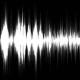### Math Brain Teaser: The Unkindest Cut of All, Part 1 of 2

In hon­or of Math­e­mat­ics Aware­ness Month, here is anoth­er math­e­mat­i­cal brain ben­der from puz­zle mas­ter Wes Car­roll.

The Unkind­est Cut of All, Part 1 of 2

Dif­fi­cul­ty: HARD
Type: MATH (Spa­tial)Ques­tion:
The area of a square is equal to the square of the length of one side. So, for exam­ple, a square with side length 3 has area (32), or 9. What is the area of a square whose diag­o­nal is length 5?

In this puz­zle you are work­ing out your spa­tial visu­al­iza­tion (occip­i­tal lobes), mem­o­ry (tem­po­ral lobes), and hypoth­e­sis gen­er­a­tion (frontal lobes).

Solu­tion:
12.5

Expla­na­tion:
I am espe­cial­ly fond of these two ways to solve this prob­lem:

1. Draw the right tri­an­gle whose hypotenuse is the square’s diag­o­nal, and whose two legs are two sides of the square. Then use the Pythagore­an The­o­rem (a^2 + b^2 = c^2) to solve for the length of each side. Since two sides are equal, we get (a^2 + a^2 = c^2), or (2(a^2) = c^2) ). Since c is 5, 2(a^2) = 25, mak­ing a^2 equal to 25/2, or 12.5. Since the area of the square is a^2, we’re done: it’s 12.5.

2. Tilt the square 45 degrees and draw a square around it such the the cor­ners of the orig­i­nal square just touch the mid­dles of the sides of the new, larg­er square. The new square has sides each 5 units long (the diag­o­nal of the small­er square), and it there­fore has area 25. How­ev­er, a clos­er inspec­tion reveals that the area of the larg­er square must be exact­ly twice that of the small­er. There­fore the small­er square has area 25/2, or 12.5.

You can now go on to Con­cen­tric Shapes: The Unkind­est Cut of All, Part 2 of 2

More brain teas­er games:

### 13 Responses

1.Omar says:

I feel the solu­tion is too long, eas­i­est thing to do is:

you know that the diag­o­nal is sq.rt(2)*x where x is the mea­sure­ment of the side. since that’s true, then swuare the answer nd you’re done. so [5/2qrt(2)]^2 = 12.5

2.Juan says:

There is anoth­er easy way:

The diag­o­nal divides the square in two tri­an­gles. Each tri­an­gle has an area of bxh/2
b = diag­o­nal
h = diag­o­nal / 2

so 5 x 2.5 / 2 = 6.25
6.25 x 2 tri­an­gles = 12.5

I think you would have to set the dif­fi­cul­ty to Easy.

Bye.

3.Hymen says:

I con­cur!

4.Mitch says:

12.5
a^2 + b^2 = c^2
You square the length of the diag­o­nal ©.
25 = a^2 + b^2
because its a square both sides are equal. So divide by 2 to get the square of 1 of the sides ie the vol­ume.

5.DJ_Dysfunk says:

Notice two diag­o­nal lines, one for each pair of oppos­ing cor­ners of the square, form four halves of 2 squares. The length of the side of any of these halves is equal to half of 5, or 2.5. The area of a square is one of its sides squared, 2.5^2 = 6.25 * 2 = 12.5, as (again) there are four halves of two squares.

6.Sharon says:

A^2+b^2=c^2. C = 5, and a =b. 5x5=25=c^2. A^2+b^2=25. a^2 and b^2 there­fore both equal 12.5. A^2 is the for­mu­la for area, so the area is 12.5

7.John says:

12.53 where the square root of 2 = 1.41421356

8.Egon_Freeman says:

I con­cur, this puz­zle’s dif­fi­cul­ty set­ting should be changed to “Easy”. I arrived at the result in about 15 sec­onds, and that’s cer­tain­ly not brag­ging…

9.alside says:

I believe the pur­pose for these puz­zles is to exer­cise the por­tions of the brain that are in use when com­plet­ing the activ­i­ty.

10.Bill says:

You guys are mak­ing this too hard.

Two isosce­les tri­an­gles with hypotenuse 25.

Each oth­er side is the square root of 12.5.

square root of 12.5 squared is 12.5

### Top 10 Brain Teasers and Illusions

1. You think you know the colors? Try the Stroop Test
2. Check out this brief attention experiment